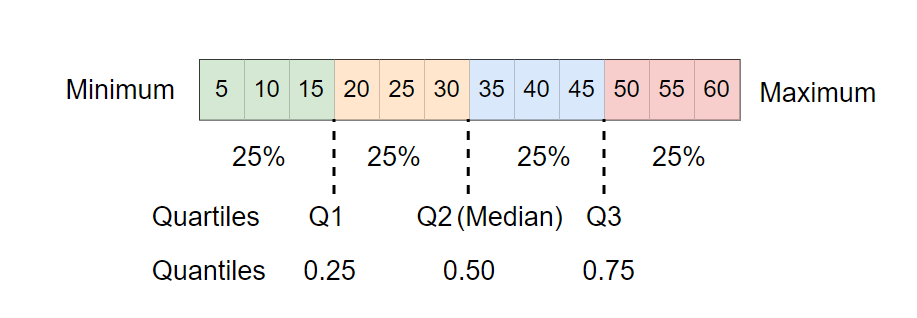# Calculate Quartiles in Python

Quartiles are values that divide a dataset into four equal parts, each containing 25% of the data. Quartiles are useful for understanding the spread and distribution of a dataset.

In general, there are three quartiles used. Q1 (first quartile), Q2 (second quartile), and Q3 (third quartile) are the values below which 25%, 50%, and 75% of the data fall.In Python, the quartiles can be calculated using the `quantile()` function from the NumPy and pandas package.

The general syntax of `quantile()` looks like this:

``````# calculate quartiles using using NumPy
import numpy as np
np.quantile(x, [0.25, 0.5, 0.75])

# calculate quartiles using pandas
import pandas as pd
df['col_name'].quantile([0.25, 0.5, 0.75])
``````

Where, `x` is the dataset in array format and the second array is the probability for the quantiles to compute.

The following examples explain how to use the `quantile()` function from NumPy and pandas to calculate quartiles

## Example 1: calculate quartiles using `quantile()` from NumPy

Suppose, you have a following dataset,

``````x = [5, 10, 15, 20, 25, 30, 35, 40, 45, 50, 55, 60]
``````

Calculate the quartiles using `quantile()` function from NumPy,

``````# import package
import numpy as np

# calculate quartiles
np.quantile(x, [0.25, 0.5, 0.75])

# output
array([18.75, 32.5 , 46.25])
``````

The Q1, Q2, and Q3 quartile values are 18.75, 32.5, and 46.25, respectively.

## Example 2: calculate quartiles using `quantile()` from pandas

Suppose, you have the following pandas DataFrame,

``````# import package
import pandas as pd

# create random pandas DataFrame
df = pd.DataFrame({'col1': ['A', 'B', 'C', 'D', 'E', 'F', 'G', 'H', 'I', 'J', 'K', 'L'],
'col2': [5, 10, 15, 20, 25, 30, 35, 40, 45, 50, 55, 60]})

# view first few rows
col1  col2
0    A     5
1    B    10

# calculate quartiles
df['col1'].quantile([0.25, 0.5, 0.75])

# output
df['col2'].quantile([0.25, 0.5, 0.75])
0.25    18.75
0.50    32.50
0.75    46.25
Name: col2, dtype: float64
``````

The output shows that Q1, Q2, and Q3 quartile values are 18.75, 32.5 , and 46.25, respectively.

## Subscribe to get new article to your email when published

* indicates required